# The Gyroelongated Square Bicupola

The gyroelongated square bicupola is the 45th Johnson solid (J45). It has 24 vertices, 56 edges, and 34 faces (24 equilateral triangles and 10 squares).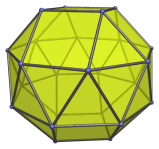The gyroelongated square bicupola can be constructed by attaching two square bicupolae to an octagonal antiprism, or equivalently, adding a second square cupola to a gyroelongated square cupola (J23).

J45 is one of the few Johnson solids that are chiral; it is distinct from its own mirror image: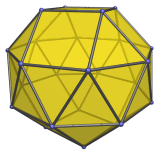## Projections

Here are some views of the gyroelongated square bicupola from various angles:

Projection Description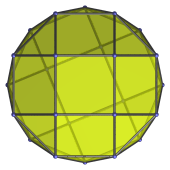Top view.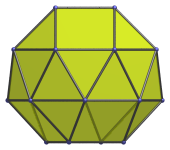Front view.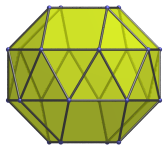11.25° side view.

## Coordinates

The Cartesian coordinates of the gyroelongated square bicupola with edge length 2 are:

• (±1, ±1, H+√2)
• (±1, ±(1+√2), H)
• (±(1+√2), ±1, H)
• (0, ±√(4+2√2), −H)
• (±√(4+2√2), 0, −H)
• (±√(2+√2), ±√(2+√2), −H)
• (√(1+1/√2), √(1−1/√2), −H−√2)
• (−√(1+1/√2), −√(1−1/√2), −H−√2)
• (√(1−1/√2), −√(1+1/√2), −H−√2)
• (−√(1−1/√2), √(1+1/√2), −H−√2)

where H = √((1+√2)(√(1+1/√2)−1)), or approximately 0.860296, is half the height of a hexagonal antiprism of edge length 2.

Last updated 03 Sep 2018.